PSA9CEP - Student-Facing Tasks---7.1 Lesson 6: Scaling and Area: Student Facing Tasks (7.G.A.1, 7.G.B.6)
Part A)

Your teacher will give you some pattern blocks. Work with your group to build the scaled copies described in each question.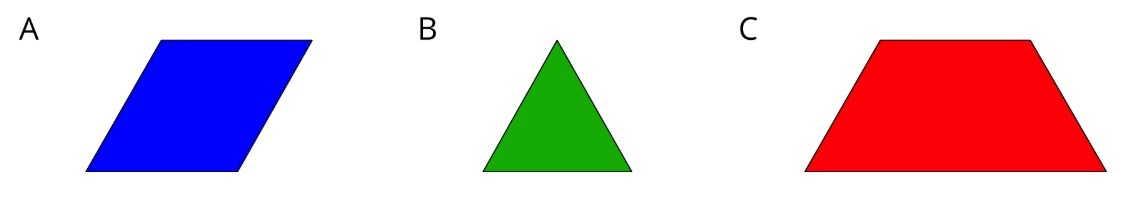How many blue rhombus blocks does it take to build a scaled copy of Figure A where each side is twice as long?

Type your answer below as a number (example: 5, 3.1, 4 1/2, or 3/2):
Part B)

How many blue rhombus blocks does it take to build a scaled copy of Figure A where each side is 3 times as long?

Type your answer below as a number (example: 5, 3.1, 4 1/2, or 3/2):
Part C)

How many blue rhombus blocks does it take to build a scaled copy of Figure A where each side is 4 times as long?

Type your answer below as a number (example: 5, 3.1, 4 1/2, or 3/2):
Part D)

How many green triangle blocks does it take to build a scaled copy of Figure B where each side is twice as long?

Type your answer below as a number (example: 5, 3.1, 4 1/2, or 3/2):
Part E)

How many green triangle blocks does it take to build a scaled copy of Figure B where each side is 3 times as long?

Type your answer below as a number (example: 5, 3.1, 4 1/2, or 3/2):
Part F)

How many green triangle blocks does it take to build a scaled copy of Figure B using a scale factor of 4?

Type your answer below as a number (example: 5, 3.1, 4 1/2, or 3/2):
Part G)

How many red trapezoid blocks does it take to build a scaled copy of Figure C using a scale factor of 2?

Type your answer below as a number (example: 5, 3.1, 4 1/2, or 3/2):
Part H)

How many red trapezoid blocks does it take to build a scaled copy of Figure C using a scale factor of 3?

Type your answer below as a number (example: 5, 3.1, 4 1/2, or 3/2):
Part I)

How many red trapezoid blocks does it take to build a scaled copy of Figure C using a scale factor of 4?

Type your answer below as a number (example: 5, 3.1, 4 1/2, or 3/2):
Part A)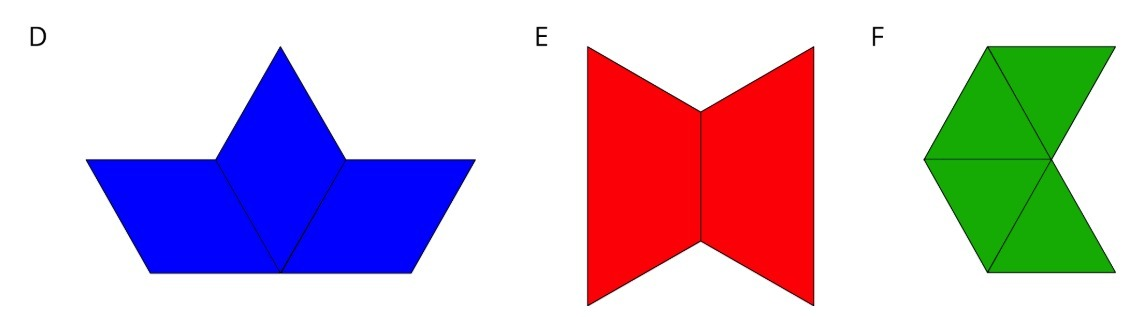Build a scaled copy of your assigned shape using a scale factor of 2. Use the same shape blocks as in the original figure. How many blocks did it take?

Part B)

Your classmate thinks that the scaled copies in the previous problem will each take 4 blocks to build. Do you agree or disagree? Explain your reasoning.

Part C)

Start building a scaled copy of your assigned figure using a scale factor of 3. Stop when you can tell for sure how many blocks it would take. Record your answer.

Part D)

How many blocks would it take to build scaled copies of your figure using scale factors 4, 5, and 6? Explain or show your reasoning.

Part E)

How is the pattern in this activity the same as the pattern you saw in the previous activity? How is it different?

Part A)

Your teacher will give you a figure with measurements in centimeters. What is the area of your figure?

Type your answer below as a number (example: 5, 3.1, 4 1/2, or 3/2):
Part B)

Your teacher will give you a figure with measurements in centimeters. What is the area of your figure?

How do you know?

Part C)

Work with your partner to draw scaled copies of your figure, using each scale factor in the table. Complete the table with the measurements of your scaled copies.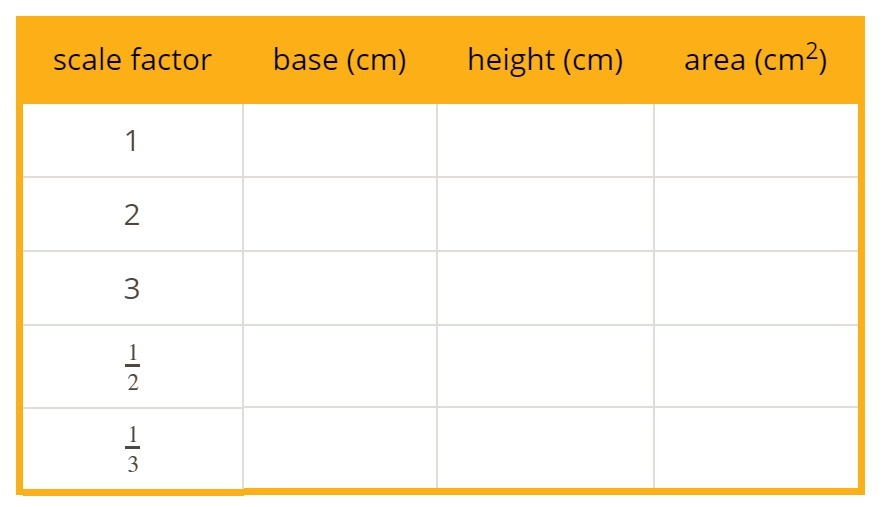Create and fill in the table using the table button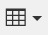Part D)

Part E)

If you drew scaled copies of your figure with the following scale factors, what would their areas be? Discuss your thinking. If you disagree, work to reach an agreement. Be prepared to explain your reasoning.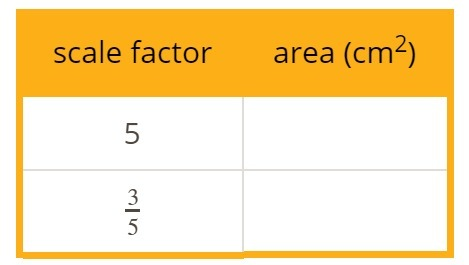Part A)Lin has a drawing with an area of 20 in2. If she increases all the sides by a scale factor of 4, what will the new area be?